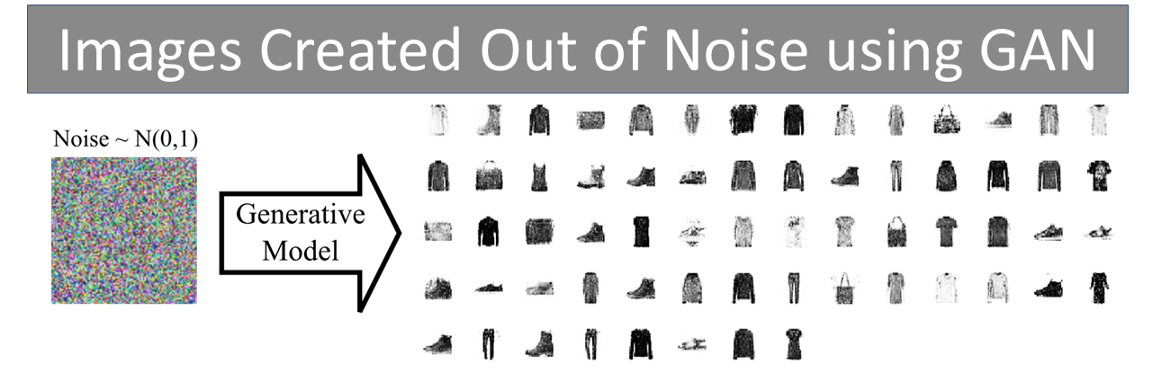# Building and training a Convolution GAN with Keras

Original article was published by Surajit Saikia on Artificial Intelligence on Medium# Building and training a Convolution GAN with Keras

In this article, we will learn how to build a GAN from scratch using Convolution layers. For demonstration and quick work out, we will be using the Fashion MNIST dataset. And after you learn it, similarly you can do for faces. Before going into details I want you to think, Can we use Deep learning to create something from nothing? To answer this question we are going to implement a GAN and see the results with our own eyes.

GAN is a unification of two Neural networks: Generator and Discriminator. The generator, generates new data instances, while the other, the discriminator, evaluates them for authenticity; i.e. the discriminator decides whether each instance of data that it reviews belongs to the actual training dataset or not. Now, let us implement and see it ourself.

1. Tensorflow ( I tested using 1.12)
2. Keras
3. Pandas
4. OpenCV

You can now start by opening a Jupyter-notebook and start typing each code snippet in your notebook.

`(x_train,y_train), (x_test, y_test) = fashion_mnist.load_data()#We next normalize the imagesx_train = (x_train.astype(np.float32) - 127.5)/127.5`

Now we have the training data available, which contains 60K images with shape (28x28x1). So, we need to create a Generator which accepts images of that particular shape.

`def create_discriminator():    discriminator=Sequential()    discriminator.add(Dense(units=512,input_dim=28*28))    #discriminator.add(Conv2D(64, (3,3), strides =(1,1), padding ='same', input_shape = [28,28,1]))    discriminator.add(LeakyReLU(0.2))    discriminator.add(Dropout(0.3))    #discriminator.add(Flatten())    discriminator.add(Dense(units=512))    discriminator.add(LeakyReLU(0.2))    discriminator.add(Dropout(0.3))    discriminator.add(Dense(units=256))    discriminator.add(LeakyReLU(0.2))    discriminator.add(Dense(units=1, activation='sigmoid'))    discriminator.compile(loss='binary_crossentropy', optimizer=adam_optimizer())    return discriminatord =create_discriminator()d.summary()#Summary of the GeneratorLayer (type)                 Output Shape              Param #    ================================================================= conv2d_3 (Conv2D)            (None, 10, 10, 64)        640        _________________________________________________________________ batch_normalization_3 (Batch (None, 10, 10, 64)        256        _________________________________________________________________ leaky_re_lu_13 (LeakyReLU)   (None, 10, 10, 64)        0          _________________________________________________________________ conv2d_4 (Conv2D)            (None, 4, 4, 32)          18464      _________________________________________________________________ batch_normalization_4 (Batch (None, 4, 4, 32)          128        _________________________________________________________________ leaky_re_lu_14 (LeakyReLU)   (None, 4, 4, 32)          0          _________________________________________________________________ flatten_3 (Flatten)          (None, 512)               0          _________________________________________________________________ dense_15 (Dense)             (None, 512)               262656     _________________________________________________________________ leaky_re_lu_15 (LeakyReLU)   (None, 512)               0          _________________________________________________________________ dense_16 (Dense)             (None, 1024)              525312     _________________________________________________________________ leaky_re_lu_16 (LeakyReLU)   (None, 1024)              0          _________________________________________________________________ dense_17 (Dense)             (None, 784)               803600     ================================================================= Total params: 1,611,056 Trainable params: 1,610,864 Non-trainable params: 192`

We have created a Generator which consists of two Convolutional layers followed by two dense layers. The final dense layer has the shape (28×28) so that we can reconstruct it back to images as in the dataset.

Next, we create the discriminator which is going to determine if the images created by the Generator are fake or Real. If the discriminator predicts those images to be fake, then the generator modifies its weight until it can cheat the discriminator. This is how the Generator is going to learn to create images out of noises.

`def create_discriminator():    discriminator=Sequential()    discriminator.add(Dense(units=512,input_dim=28*28))    #discriminator.add(Conv2D(64, (3,3), strides =(1,1), padding ='same', input_shape = [28,28,1]))    discriminator.add(LeakyReLU(0.2))    discriminator.add(Dropout(0.3))    #discriminator.add(Flatten())    discriminator.add(Dense(units=512))    discriminator.add(LeakyReLU(0.2))    discriminator.add(Dropout(0.3))    discriminator.add(Dense(units=256))    discriminator.add(LeakyReLU(0.2))    discriminator.add(Dense(units=1, activation='sigmoid'))    discriminator.compile(loss='binary_crossentropy', optimizer=adam_optimizer())    return discriminatord =create_discriminator()d.summary()`

We have created both the Generator and the Discriminator. Next, we unify both of them to create the GAN. In the code below, we unify the two modules and we train them.

`def create_gan(discriminator, generator):    discriminator.trainable=False    img_shape=(28, 28, 1)    gan_input = Input(img_shape)    x = generator(gan_input)    gan_output= discriminator(x)    gan= Model(inputs=gan_input, outputs=gan_output)    gan.compile(loss='binary_crossentropy',     optimizer=adam_optimizer())    return gan`

Now let us train the GAN by unifying Generator with the Discriminator

`generator= create_generator()discriminator= create_discriminator()gan = create_gan(discriminator, generator)batch_size = 128Epochs = 300for epcs in range(1, Epochs ):        print("Epoch Number %d" %epcs)        for _ in tqdm(range(batch_size)):        #generate  random noise of shape 28*28            noise= np.random.normal(0,1, [batch_size, 28,28,1])            # Now predict the noise by feeding it to generator            generated_images = generator.predict(noise)            # Take real images from the Fashion MNIST            image_batch =x_train[np.random.randint                        (low=0,high=x_train.shape,size=128)]            # The discriminator accepts vector of dimension 784            image_batch = image_batch.reshape(-1,28*28)            #We concate both real and fake images             X= np.concatenate([image_batch, generated_images])            # We Next assign label to X            y_discriminator=np.zeros(2*batch_size)            # First  images are true, so we label them 1            y_discriminator[:batch_size]=1            #Pretrain discriminator on  fake and real data             discriminator.trainable=True            discriminator.train_on_batch(X, y_discriminator)            # We trick the noises to be real            noise= np.random.normal(0,1, [batch_size, 28,28,1])            y_label = np.ones(batch_size)            discriminator.trainable=False            # Let us train the GAN              gan.train_on_batch(noise, y_label)`

After the training is over you can predict the Generator based on the noise.

`# feed the noises to the generator and predict themgenerated_images = generator.predict(noise)#we are going to see the first image i.e at index 0x =generated_images.reshape(28,28)# Let us plot the imageplt.imshow(x, cmap ="Greys")`

Below are the images generated out of noises, and now you can start testing on your own images.